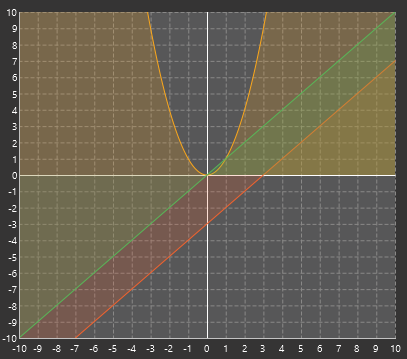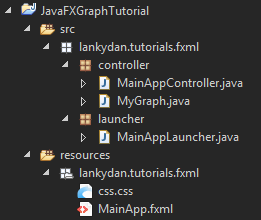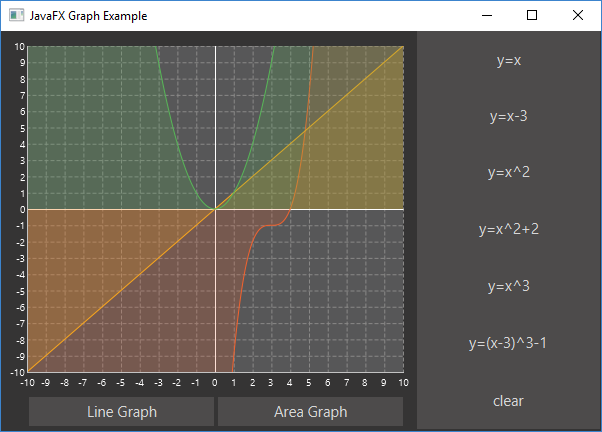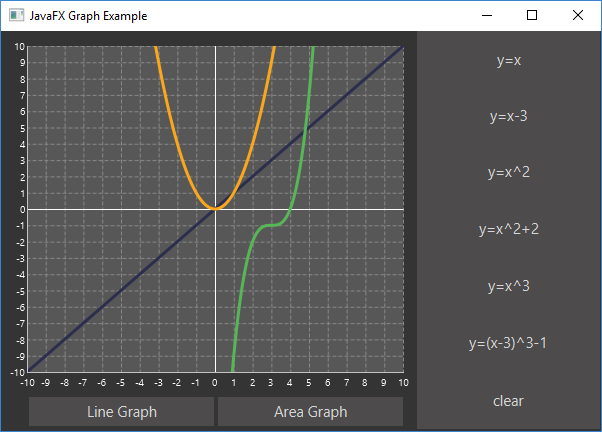# JavaFX Charts Look Pretty Good!

### With some tweaking, you can get JavaFX to compile nice-looking charts with a few animations baked in. Configuring them takes a bit of work, but it's worth it.

· Java Zone · Tutorial
Save
29.33K Views

When I was doing my third-year project in university, I needed a good-looking chart to use in my user interface. Now that I have grown older (nearly two whole years!), I wish I would have turned to the charts in JavaFX rather than the ugly looking JChart2D API that I used. Now don’t get me wrong, I’m not hating on JChart2D, as it was easy to use and, at the time, I was really happy with it. But the charts/graphs that you can use in JavaFX look really good, and they even come with some fancy animations by default. Okay, I’m finished hyping up these graphs, so I’m going to show you a little example application I wrote, and, hopefully, you will feel the same about them as me.Before you go any further, I recommend that you read up on the basics of using JavaFX. My other post Making Apps with JavaFX covers this.

In this post, I will cover the basic use of a LineChart and AreaChart. The code is pretty simple and only requires a little bit of set up in the Controller and fxml. All you need to do is add the chart to the fxml code, give it a fx:id, set up its properties to your liking, and you're done.

``````<LineChart fx:id="lineGraph" createSymbols="false" legendVisible="false" prefHeight="372.0" prefWidth="423.0" visible="false">
<xAxis>
<NumberAxis autoRanging="false" lowerBound="-10" side="BOTTOM" tickUnit="1" upperBound="10" />
</xAxis>
<yAxis>
<NumberAxis autoRanging="false" lowerBound="-10" side="LEFT" tickUnit="1" upperBound="10" />
</yAxis>
<cursor>
<Cursor fx:constant="CROSSHAIR" />
</cursor>
</LineChart>``````

This is this the setup for the LineChart. It defines the axis’ upper and lower bounds, which will change the chart to look more like a graph. By setting the lower bound of the x or y axis, it will cause the chart to auto draw lines to represent the axis that will pass through the coordinates (0,0). The tickUnit represents how separated the grid lines on the chart are and autoRanging has also been disabled to prevent the chart from resizing itself to best fit the content that is on the chart. Finally, a cursor has been defined. There are a few other cursors that can be used, but I think the crosshair cursor looks the best.

Now that you know how to create the chart, you're going to need to know how to plot some points onto it (unless you are happy with it being empty).

``````public void plotLine() {
final XYChart.Series<Double, Double> series = new XYChart.Series<Double, Double>();
for (double x = -range; x <= range; x = x + 0.01) {
}
}``````

Here, we are creating a new series object, which will contain the plotted points.

``final XYChart.Series<Double, Double> series = new XYChart.Series<Double, Double>()``

Then, adding the plots to the series.

``series.getData().add(new XYChart.Data<Double, Double>(x, Math.pow(x, 2)))``

The plotted coordinates are stored in the form of (x,y), so in the example above, the x coordinate has the value x and the y coordinate takes the value of x^2.

The final step is to add the series to the chart.

``graph.getData().add(series)``

After this has been done, the points will be plotted onto the chart and will be connected together between each plot. The order that the plots (XYChart.Data) are added to the series does not matter, as it will connect the closest plots to each other, although this isn’t relevant if you're adding plots from loops.

Now that the basics of using these charts is covered, let's get onto a larger example. Below is all the code you need to get set up.

``````public class MainAppLauncher extends Application {

public static void main(String[] args) {
Application.launch(MainAppLauncher.class, args);
}

@Override
public void start(Stage stage) throws Exception {
try {
.getResource("lankydan/tutorials/fxml/MainApp.fxml"));
stage.setScene(new Scene(root));
stage.setTitle("JavaFX Graph Example");
stage.show();
} catch (Exception e) {
System.out.print(e);
}
}
}``````

MainAppLauncher is used to load run the JavaFX code.

``````public class MainAppController implements Initializable {

@FXML
private LineChart<Double, Double> lineGraph;

@FXML
private AreaChart<Double, Double> areaGraph;

@FXML
private Button lineGraphButton;

@FXML
private Button areaGraphButton;

@FXML
private Button xyButton;

@FXML
private Button xyButton2;

@FXML
private Button squaredButton;

@FXML
private Button squaredButton2;

@FXML
private Button cubedButton;

@FXML
private Button cubedButton2;

@FXML
private Button clearButton;

private MyGraph mathsGraph;
private MyGraph areaMathsGraph;

@Override
public void initialize(final URL url, final ResourceBundle rb) {
mathsGraph = new MyGraph(lineGraph, 10);
areaMathsGraph = new MyGraph(areaGraph, 10);
}

@FXML
private void handleLineGraphButtonAction(final ActionEvent event) {
lineGraph.setVisible(true);
areaGraph.setVisible(false);
}

@FXML
private void handleAreaGraphButtonAction(final ActionEvent event) {
areaGraph.setVisible(true);
lineGraph.setVisible(false);
}

@FXML
private void handleXYButtonAction(final ActionEvent event) {
plotLine(x -> x);
}

private void plotLine(Function<Double, Double> function) {
if (lineGraph.isVisible()) {
mathsGraph.plotLine(function);
} else {
areaMathsGraph.plotLine(function);
}
}

@FXML
private void handleXYButton2Action(final ActionEvent event) {
plotLine(x -> x - 3);
}

@FXML
private void handleSquaredButtonAction(final ActionEvent event) {
plotLine(x -> Math.pow(x, 2));
}

@FXML
private void handleSquaredButton2Action(final ActionEvent event) {
plotLine(x -> Math.pow(x, 2) + 2);
}

@FXML
private void handleCubedButtonAction(final ActionEvent event) {
plotLine(x -> Math.pow(x, 3));
}

@FXML
private void handleCubedButton2Action(final ActionEvent event) {
plotLine(x -> Math.pow(x - 3, 3) - 1);
}

@FXML
private void handleClearButtonAction(final ActionEvent event) {
clear();
}

private void clear() {
if (lineGraph.isVisible()) {
mathsGraph.clear();
} else {
areaMathsGraph.clear();
}
}
}``````

MainAppController is the centerpiece of this code, which controls the chart by handling the ActionEvents fired by button presses, which, in this example, leads to the lines being drawn onto the chart. I have also used some lambda expressions in this example to make the code shorter and a little bit fancier.

``````public class MyGraph {

private XYChart<Double, Double> graph;
private double range;

public MyGraph(final XYChart<Double, Double> graph, final double range) {
this.graph = graph;
this.range = range;
}

public void plotLine(final Function<Double, Double> function) {
final XYChart.Series<Double, Double> series = new XYChart.Series<Double, Double>();
for (double x = -range; x <= range; x = x + 0.01) {
plotPoint(x, function.apply(x), series);
}
}

private void plotPoint(final double x, final double y,
final XYChart.Series<Double, Double> series) {
}

public void clear() {
graph.getData().clear();
}
}``````

MyGraph is a wrapper object that takes in an XYChart, which both LineChart and AreaChart are, and has some methods to plot and clear lines. This code could be in MainAppController, as there isn’t much code in it, but it looks much tidier like this. It uses the plotLine code shown above, although it is split out into a two methods instead.

``````<?xml version="1.0" encoding="UTF-8"?>

<?import javafx.scene.Cursor?>
<?import javafx.scene.chart.AreaChart?>
<?import javafx.scene.chart.LineChart?>
<?import javafx.scene.chart.NumberAxis?>
<?import javafx.scene.control.Button?>
<?import javafx.scene.layout.AnchorPane?>
<?import javafx.scene.layout.VBox?>

<AnchorPane maxHeight="400.0" maxWidth="600.0" minHeight="400.0" minWidth="600.0" prefHeight="400.0" prefWidth="600.0" styleClass="root" stylesheets="lankydan/tutorials/fxml/css.css" xmlns="http://javafx.com/javafx/8.0.111" xmlns:fx="http://javafx.com/fxml/1" fx:controller="lankydan.tutorials.fxml.controller.MainAppController">
<children>
<AnchorPane layoutX="416.0" minHeight="0.0" minWidth="0.0" prefHeight="400.0" prefWidth="185.0">
<children>
<VBox prefHeight="398.0" prefWidth="183.0">
<children>
<Button mnemonicParsing="false" onAction="#handleXYButtonAction" prefHeight="66.0" prefWidth="193.0" text="y=x" fx:id="xyButton" />
<Button mnemonicParsing="false" onAction="#handleXYButton2Action" prefHeight="66.0" prefWidth="207.0" text="y=x-3" fx:id="xyButton2" />
<Button fx:id="squaredButton" mnemonicParsing="false" onAction="#handleSquaredButtonAction" prefHeight="67.0" prefWidth="220.0" text="y=x^2" />
<Button fx:id="squaredButton2" mnemonicParsing="false" onAction="#handleSquaredButton2Action" prefHeight="67.0" prefWidth="232.0" text="y=x^2+2" />
<Button fx:id="cubedButton" mnemonicParsing="false" onAction="#handleCubedButtonAction" prefHeight="67.0" prefWidth="236.0" text="y=x^3" />
<Button fx:id="cubedButton2" mnemonicParsing="false" onAction="#handleCubedButton2Action" prefHeight="67.0" prefWidth="266.0" text="y=(x-3)^3-1" />
<Button fx:id="clearButton" mnemonicParsing="false" onAction="#handleClearButtonAction" prefHeight="67.0" prefWidth="266.0" text="clear" />
</children>
</VBox>
</children>
</AnchorPane>
<AnchorPane layoutX="-7.0" prefHeight="400.0" prefWidth="420.0">
<children>
<LineChart fx:id="lineGraph" legendVisible="false" prefHeight="372.0" prefWidth="423.0" visible="false">
<xAxis>
<NumberAxis autoRanging="false" lowerBound="-10" side="BOTTOM" tickUnit="1" upperBound="10" />
</xAxis>
<yAxis>
<NumberAxis autoRanging="false" lowerBound="-10" side="LEFT" tickUnit="1" upperBound="10" />
</yAxis>
<cursor>
<Cursor fx:constant="CROSSHAIR" />
</cursor>
</LineChart>
<AreaChart fx:id="areaGraph" legendVisible="false" prefHeight="372.0" prefWidth="423.0">
<xAxis>
<NumberAxis autoRanging="false" lowerBound="-10" side="BOTTOM" tickUnit="1" upperBound="10" />
</xAxis>
<yAxis>
<NumberAxis autoRanging="false" lowerBound="-10" side="LEFT" tickUnit="1" upperBound="10" />
</yAxis>
<cursor>
<Cursor fx:constant="CROSSHAIR" />
</cursor>
</AreaChart>
<Button fx:id="lineGraphButton" onAction="#handleLineGraphButtonAction" layoutX="35.0" layoutY="366.0" mnemonicParsing="false" prefHeight="29.0" prefWidth="185.0" text="Line Graph" />
<Button layoutX="224.0" onAction="#handleAreaGraphButtonAction" layoutY="366.0" mnemonicParsing="false" prefHeight="29.0" prefWidth="185.0" text="Area Graph" />
</children>
</AnchorPane>
</children>
</AnchorPane>``````

MainApp.fxml contains all the code to set up the visuals of the application.

``````#pane, .root{
-fx-background-color: #353434;
-fx-foreground-color: #353434;
}

.default-color0.chart-series-line { -fx-stroke: #2c2e4e; }

.chart-vertical-zero-line {
-fx-stroke: white;
}
.chart-horizontal-zero-line {
-fx-stroke: white;
}

.chart-plot-background {
-fx-background-color: #575758;
-fx-foreground-color: white;
-fx-stroke: white;
}

.chart-vertical-grid-lines {
-fx-stroke: #898887;
}
.chart-horizontal-grid-lines {
-fx-stroke: #898887;
}
.chart-alternative-row-fill {
-fx-fill: transparent;
-fx-stroke: transparent;
-fx-stroke-width: 0;
}

.axis {
-fx-stroke: white;
-fx-fill: white;
-fx-font-size: 1.4em;
-fx-tick-label-fill: white;
-fx-font-family: Tahoma;
-fx-tick-length: 0;
-fx-minor-tick-length: 0;
}

.background {
-fx-background-color: #674A44;
-fx-foreground-color: #353434;
}

.button {
-fx-border-color: #353434;
-fx-border-width: 0;
-fx-background-color: derive(#353434,20%);
-fx-font-family: "Segoe UI", Helvetica, Arial, sans-serif;
-fx-font-size: 11pt;
-fx-text-fill: #d8d8d8;
-fx-background-insets: 0 0 0 0, 0, 1, 2;
}

.button:hover {
-fx-background-color: #3a3a3a;
}

.button:pressed, .button:default:hover:pressed {
-fx-background-color: #bdbcbc;
-fx-text-fill: black;
}

.button:disabled, .button:default:disabled {
-fx-opacity: 0.4;
-fx-background-color: #353434;
-fx-text-fill: white;
}

.button:default {
-fx-background-color: -fx-focus-color;
-fx-text-fill: #ffffff;
}

.button:default:hover {
-fx-background-color: derive(-fx-focus-color,30%);
}

.text-area .content {
-fx-background-color: #575758;
}``````

Css.css is the stylesheet that is used for this application and is referenced inside MainApp.fxml.

If you try to run this by yourself, there are a few changes you will need to remember to make. Otherwise, you are going to run into some errors. Make sure that you have your files structured well or understand how the paths to the files work. Below is a picture of how I structured my files.Once you have sorted out your project structure, remember to rename the paths in your code to match the structure and names you have chosen. This includes the path in MainAppLauncher and the paths to controller and css in MainApp.fxml.

Once everything has been put together correctly, you should be able to run it, and it will look like this.And by pressing the Line Graph button, it will look like this.So now that you have seen a little bit of what the charts in JavaFX have to offer, you might consider using it when your writing some of your own applications. They are pretty easy to set up and come with some decent styles and animations by default. I will write a few more posts on how to use some of the other charts in the future.

Topics:
java, javafx, charts, tutorial

Published at DZone with permission of Dan Newton, DZone MVB.

Opinions expressed by DZone contributors are their own.